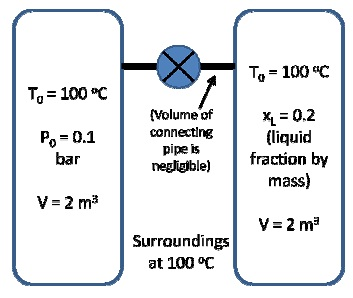How to calculate solutions molar conductance?

The contribution of an electrolyte, or an ion electrolyte, is reported as the molar of a conductance.

The definition of the molar conductance is based on the following conductivity cell in which the electrodes are 1 m apart and of sufficient area that the cell holds the amount of solution that contains 1 mol of solute. The conductivity of such a cell is the mole conductance.

A of solution of concentration c, expressed in moles per litre, has a volume in litres per mole of 1/c or a volume in cubic meters of (10-3 m3 l-1)/c. a cell with this volume and electrodes separated by 1 m would be equilivalent to (10-3 m3 l-1)/c unit cells placed alongside each other. The conductivity of such a cell, which is the molar conductance, is given by:

A = 10-3 m3 l-1/c × k

This relation defines the molar conductance in terms of the specific conductance. The concept of the cell holding solution of volume (10-3 m3 l-1)/c is introduced only to suggest the definition of conductance and in practice one uses any convenient conductance cell, measures R, and calculate L = 1/R. with this datum one obtains k= (cell constant) L and finally A.

Many precise measurements of molar conductance were made by Friedrich Kohlausch and his coworkers between about 1860 and 1880.

On the basis of such data and in the absence of any satisfactory theory about the nature of conduction in these solutions, some variable empirical relations were concluded. It was recognized that for some electrolytes plotting the molar conductance of an electrolyte at a fixed temperature against the square root of the concentration led to the plots which confirmed very closely at the lower concentrations to straight lines. Such plots for new electrolytes are lead to essentially linear plots are now classed as strong electrolytes, and those which seem to approach the dilute solution limit almost tangentially are classed as weak electrolytes.

An important relation can be deduced from extrapolations of the strong electrolyte data to infinite dilution to give what are known as limiting molar of the independent migration of ions. The law is more easily stated and understandable if some later ideas are anticipated and the conductance of an electrolyte at infinite dilution is treated as being made of contributions from the individual ions of the electrolyte. Let v+ be the number of positive ions and v - the number of negative ions implied by the formula of the electrolyte.

Molar conductances ? in Ω-1 m2 mol-1 in aqueous solution at 25° C (values for c = 0obtained by extrapolation or, for HAc and NH4OH, by a combination of extrapolated values):

 c NaCl KCl HCl NaAc CuSO4 H2SO4 HAc NH4OH 0.000 (0.012645) 0.014986 0.042616 0.00910 0.02661 0.08592 0.03907 0.002714 0.0005 (0.012450) 0.014781 0.042274 0.00892 0.02304 0.08262 0.00677 0.0047 0.001 0.012374 0.014695 0.042136 0.00885 0.01666 0.07990 0.00492 0.0034 0.010 0.011851 0.014127 0.041200 0.008376 0.01010 0.06728 0.00163 0.00113 0.100 0.010674 0.012896 0.039132 0.007280 0.00586 0.05016 0.00036 1.00 0.01119 0.03328 0.00491

#### Related Questions in Chemistry

• ##### Q :Describe various systems for

Common system According to this system, the individual members are named according to alkyl groups att

• ##### Q :Means of molal solution Choose the

Choose the right answer from following. A molal solution is one that contains one mole of a solute in: (a) 1000 gm of the solvent (b) One litre of the solvent (c) One litre of the solution (d) 22.4 litres of the solution

• ##### Q :Relative lowering of the vapour pressure

Choose the right answer from following.The relative lowering of the vapour pressure is equal to the ratio between the number of: (a) Solute moleules and solvent molecules (b) Solute molecules and the total molecules in the solution (c) Solvent molecules and the tota

• ##### Q :Hybridization Atomic orbitals can be

Atomic orbitals can be combined, in a process called hybridization, to describe the bonding in polyatomic molecules. Descriptions of the bonding in CH4 can be used to illustrate the valence bond procedure. We must arrive a

• ##### Q :Explain Rotational Vibrational Spectra

The infrared spectrum of gas samples shows the effect of rotational-energy changes along with the vibrational energy change.As we know from the interpretations given to thermodynamic properties of gases, gas molecules are simultaneously rotating and vibrating. It follows that an absor

• ##### Q :Problem on vapor-liquid equilibrium Two

Two tanks which contain water are connected to each other through a valve. The initial conditions are as shown (at equilibrium):• ##### Q :Amines arrange in order of

arrange in order of basicity,pyridine,pipyridineand pyorine

• ##### Q :Problem on equilibrium constant Ethanol

Ethanol is manufactured from carbon monoxide and hydrogen at 600 K and 20 bars according to the reaction2 C0(g) + 4 H2(g) ↔ C2H5OH(g) + H2O (g)The feed stream contains 60 mol% H2, 20 m

• ##### Q :Atmospheric pressure Give me answer of

Give me answer of this question. The atmospheric pressure is sum of the: (a) Pressure of the biomolecules (b) Vapour pressure of atmospheric constituents (c) Vapour pressure of chemicals and vapour pressure of volatile (d) Pressure created on to atmospheric molecules

• ##### Q :Molarity based question Help me to

Help me to solve this problem. 4.0 gm of NaOH are contained in one decilitre of solution. Its molarity would be: (a) 4 M (b)2 M (c)1 M (d)1.5 M